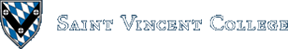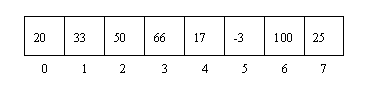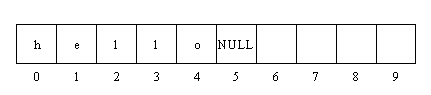Computing & Information Systems    DepartmentSearch CIS Site      Tutorials

## Software Design Using C++

### Introduction

In this Web page we will look at the simplest kinds of arrays. Thus we will only look at 1-dimensional arrays and won't do any complicated processing with them. For more advanced information on arrays, look at the intermediate section on arrays in these Web pages.

A 1-dimensional array is used to hold a sequence of items all of the same type. An index number is used to specify each storage location in the array. In C++ indexing begins with 0. For an array named A, the first storage location is A, the second location is A, etc. An array of 8 integers would look like this:Let's assume that the name of this array is A. We could place the 20 into array location 0 by using the following assignment statement:

 `````` A = 20; ``````

We could read a number into an array location, also. For example, to read in a number into array location 1 we might use something like this:

 `````` cout << "Enter a number:"; cin >> A; ``````

Since we often want to place numbers into all of the array locations, we are likely to write a loop to handle things. In many cases we know the number of items to be processed, so that a FOR loop is typical. Here is an example of a FOR loop that reads numbers into all 8 locations of our array A. This is followed by another FOR loop that writes out all of the data in array A.

 `````` int k; for (k = 0; k < 8; k++) { cout << "Enter an integer: "; cin >> A[k]; } for (k = 0; k < 8; k++) cout << A[k] << endl; ``````

### Array of Integers Example

Look at array1.cpp for a more interesting example using an array of integers. This program asks the user how many numbers are to be entered, then reads that many numbers into an array. Finally, the program finds and prints the average of the numbers.

At the top of this program you find the following code to set up one constant and one new type. `ArrayMax` is set up as an integer constant with value 10. This is used as the size of our array. Then we see something new, a `typedef`. It is used to set up a new type, here called `ArrayType`, that stands for an array of 10 integers. Note that a type name is different than a variable name. `ArrayType` is not a variable at all. It is just the type information that could be used for a variable or parameter. Thus `ArrayType` is much like the built-in types `int`, `float`, etc.

 `````` const int ArrayMax = 10; typedef int ArrayType[ArrayMax]; ``````

Although not absolutely necessary, it is a great convenience to have a one word name for such a type. We will consistently use a `typedef` for every array type throughout these Web pages. Note that our new type is used to give the type information for the `NumArray` parameter in the `FillArray` function.

 `````` void FillArray(ArrayType NumArray, int & Count); ``````

If you read the comment section for this function, you will see that it returns data in both parameters. Normally a parameter must be a reference parameter, the kind that uses an ampersand, in order to return an answer. Why, then, is there no ampersand on the `NumArray` parameter? That is because arrays in C++ are a kind of automatic reference parameter. Do not use an ampersand on an array parameter.

The main function creates an array of type `ArrayType` as follows. This is exactly the same syntax as we used for the array parameter above.

 `````` ArrayType MyArray; ``````

The bulk of the work in the main function consists in calling the two helping functions, as shown below. The `FillArray` function is used to place data into `MyArray` and to place the number of items into `NumItems`. If you read the code for this function, you will see that it uses a straightforward FOR loop as discussed in the introduction above. The `FindAverage` function is passed the array and `NumItems` for use in calculating the average. The average itself is returned in the function name and assigned into the variable `Avg` as shown.

 `````` FillArray(MyArray, NumItems); Avg = FindAverage(MyArray, NumItems); ``````

Note how these functions are commented with the "Given, Task, Return" method. Any values passed into a function are listed, by name, and described in the "Given" section. A parameter that contains only garbage data when the function is called (such as `NumItems` in the `FillArray` function) is not listed in the "Given" section. Any values passed back out of the function are listed in the "Return" section, either identified by parameter name or described as the value passed back in the function name if that is the mechanism being used. Output to the screen is not considered to be a return value and is thus not mentioned in the "Return" section.

The bulk of the code for the `FindAverage` function is pasted in below for your examination. The loop shows the usual way to calculate a total: start a running sum at zero and add on one new number after another. Here the numbers to add on are taken from the array, each time at one larger index.

 `````` Sum = 0.0; for (k = 0; k < Count; k++) Sum = Sum + NumArray[k]; if (Count > 0) return Sum / Count; else return 0.0; ``````

The `if` shown here is a typical example of how to skip something. In this case, we mostly want to avoid dividing by zero, which is not an allowed operation in algebra! This is sometimes called a "skip guard" plan. The code also shows you that it is legal to have more than one `return` statement in a function. As soon as a `return` statement is executed, the function is exited and the value returned is sent back to whatever called this function.

### A Better Average Program

Now, just because the above program works doesn't mean that it is a reasonable way to find the average. In fact it is unreasonable. There is no need to save all of those numbers up in an array when we could have just added them up as the user entered them. Then the average could be found as this sum divided by the number of items. What could be simpler? (A good general rule is to never use a complex method when a simpler one is available.) Our program above wastes space by needlessly storing numbers in an array. In some applications the number of items might be huge so that an array would waste a lot of space. Don't use arrays unless there is a good reason to save all of that data!

A more sensible program to handle the average is array2.cpp. It uses the approach described above of just summing the numbers as they are entered. The numbers themselves are not saved. There is no need for an array, and the code is so short that it can easily all be placed into the main function without having things look too complicated.

### Where an Array is Needed

When would we need to save up data in an array? Basically whenever we need to look at the data more than once. The program array4.cpp is such a case. In this program we enter some integers and average them, that's one pass over the data. But after finding the average we want to print out the numbers that are below this average. That requires a second pass over this data. There is no way to print the below average numbers until you know what the average is! An array is just the thing needed to save the data for that second pass.

This program is much like our older one. Essentially we have just added a new helping function to print out the below average numbers and called it at the appropriate place in the main function. Dividing things up into functions, each of which handles one main task, helps when adding new functionality or when adding the same kind of thing to a new program. The bulk of the code for our new function is shown below:

 `````` for (k = 0; k < Count; k++) { if (NumArray[k] < Average) cout << NumArray[k] << endl; } ``````

The main thing in here is the comparison of a number from the array with the average. Each time around the loop the index `k` is one larger. That way we are testing a different number from the array each time around.

### Array of Characters (a String)

An array of characters is a simple way to implement a string, something that could hold someone's name or other text data. Safer and more advanced ways to handle strings can be used once you have some experience with using objects. (See the STL string class as well as the discussion of buffer overflows and safely copying string with the STL string class.) When implementing a string as an array of characters, the array just holds one character after another, starting at index 0. After the last character of data, a NULL character is placed in the next array location. It does not matter if there are unused array locations after that. They will simply contain garbage data.

A NULL character is a special character (represented by the ASCII code 0) used to mark the end of this type of string. A literal character array is written in double quotes, as in "hello". These have been used before as messages in our output statements. A picture of the array holding "hello" would be as follows, assuming that the array is of length 10:We need to know how to get data into a character array, and how to print it. Those are the two basic operations that we will learn here. For more advanced information on characters arrays, look at the characters arrays topic within the intermediate page on arrays.

For our purposes here, we will use the array3.cpp example. Toward the top of this program you see that we have set up one constant and one type. `MaxString` is set up as an integer constant containing the value 32. The `typedef` sets up a new type called `StringType` that is an array of 32 characters.

 `````` const int MaxString = 32; typedef char StringType[MaxString]; ``````

Since this program is short, the code for the main function has been copied in below, in slightly simplified form. Note how you create a character array variable: you simply say it has type `StringType`. You can optionally give the variable a value by using = followed by a literal string in double quotes, as is done below with `ErrMsg`. Note that this is the only place where you can "assign" a string into a character array. You cannot do this later in the code, after the declaration of the variable.

 `````` StringType UserName; StringType ErrMsg = "An error has been encountered"; cout << "Enter your complete name: "; cin.getline(UserName, MaxString); cout << "User name entered was: " << UserName << endl; cout << "If an error happened we could print this message:"; cout << endl << ErrMsg << endl; ``````

So, one way to get a string into a character array is to place it there when you declare the variable. However, from what was said above, it would be illegal to try to assign it into the variable after the declaration. Thus the following is not allowed:

 `````` StringType ErrMsg; ErrMsg = "An error has been encountered"; ``````

Another common way to get a string into a character array is to read it in. The program above uses the `getline` function to do this. This is actually a class function that is being applied to the `cin` object. You will learn more about objects later. For the moment, just realize that the `cin.getline` syntax means that the `getline` function is somehow being applied to the `cin` "stream". The `cin` stream is used for reading data from the keyboard. You will learn more about streams later in the Intermediate section of these web pages. This call of the `getline` function reads up to `MaxString - 1` characters into `UserName`, tacks on the NULL to mark the end of the string, and throws away the newline generated when the user presses ENTER after typing the string. You might ask why we don't use the following to read in a string:

 `````` cin >> UserName; ``````

This works to some extent. However, it stops at the first blank space. Since there is blank space between a person's first and last name, this is not a good method for reading a name. The `getline` function is better behaved in this regard; it reads in blank space with no problem. Still, we can have some difficulties with `getline`. We could, for example, try to read in more characters than will fit in the array. That will definitely cause a problem. (For more information on this, look at the characters arrays topic within the intermediate page on arrays.)

Printing out a string stored in a character array is very easy. You just output it the same way you would output the value of an integer or float variable. The last few lines of our example program illustrate this.